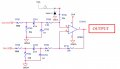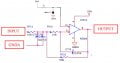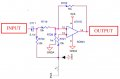# Envelope decoder circuit - can that be changed?

#### morfeus

Joined Nov 18, 2012
4
Hi everyone,

I would like to make an envelope decoder circuit similar as described in an NXP application note named AN4701, that can be found here:
https://www.nxp.com/docs/en/application-note/AN4701.pdf
However, I would like to have much higher signal input (up to 150V) so I have to change some things (while given descriptions are, at least how I can understand it, for maximum 40V pk-pk (by the description on page 7, chapter "3.1. Voltage scale down circuit"). So, I have this 5 things and questions about the designs:
1. Page 6: "Figure 7. Voltage scale down circuit."
R701-R703 and R702-R704 make voltage divider. For 150V, this shall be changed to:
R701: 390k
R703: 15k
R702: 390k
R704: 24k
2. Page 6: "Figure 7. Voltage scale down circuit."
As I understand, 5VRef with resistors R708 and R707 make a voltage divider that makes 2.5V on "upper" connection point of the resistor R709. Can that just be changed with an LM4040-2.5 as a 2.5V reference voltage? If yes, what current to take in formula for Rs?3. Page 8: "Figure 11. Low-pass filter"
Here I would also change 5VRef, R717, R716 with LM4040-2.5 and Rs combination like described under 2. If that's possible, Can LM4040-2.5 and Rs be "shared" with "Voltage scale down" circuit?4. Page 11: "Figure 14. Comparator circuit"
Here I would also change 5VRef, R723, R724 with LM4040-2.5 and Rs combination like described under 2. If that's possible, Can LM4040-2.5 and Rs be "shared" with "Voltage scale down" circuit?
5. Page 11: "Figure 14. Comparator circuit"
Is R728 really 1mOhm (miliohm) or this is some kind of error in the design?Sorry for the image quality and "photoshoping" but I still draw my circuit by hand on paper and then put all in CAD (and this images are far far better to see than my hand-drawing).Thanks for any help!

Kind regards,
Gabrijel